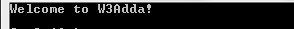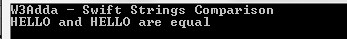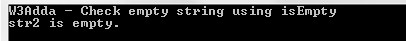Swift Strings

In this tutorial you will learn about the Swift Strings and its application with practical example.

Swift Strings

String is a series or sequence of characters, a string variable is used to hold string value that could be consists of letters, numbers, and any special characters.

Example:-

Below is a list of some valid string –

Declaring Strings In Swift

In Swift, a strings can be declared using double quotes “Hello World”.

Syntax:-

Example:-

Output:-

Swift Strings Concatenation

In Swift, strings can be concatenated simply using the plus (‘+’) operator or the += assignment operator.

Example:-

When you will run above code, you will see the following output.

Output:-

If you want to append a characters to any string it can be simply done using append() method as following –

Example:-

Output:-Swift Strings Comparison

In swift, equal to (==) and not equal (!=) operators can used to check whether the given two strings are equal or not.
The equality operators will perform case-sensitive comparison which means “HELLO” and “hello” are not equal.

Example:-

When you will run above code, you will see the following output.

Output:-Swift Check Empty Strings

In swift, isEmpty property can be used check if a given string is empty string or not.

Example:-

When you will run above code, you will see the following output.

Output:-In this tutorial we have learn about the Swift Strings and its application with practical example. I hope you will like this tutorial.